Practice Calculus Problems for the AP Calculus AB Exam

There is no such thing as doing too many practice calculus problems when studying for the AP exam. It is important no matter what stage in AP Calculus preparation you are at. Going through practice problems will remind you of the possible topics that could be on the exam. It helps you gain confidence since many similar problems repeat themselves year after year (not the same problem, but similar types of problems). And learning the subject in theory, without ever working through examples, is rarely enough to truly understand how concepts can be implemented. The absolute best sources for problems is actual past AP exams. The College Board provides a few older exams, and you can buy College Board books that have others as well.

Practice Calculus Problems

Below is a smattering of different types of problems from across the AP Calculus AB curriculum. You need to be familiar with these concepts for the multiple choice and free response sections of the exam. A calculator is not needed for any of these problems.

Calculus Practice Problems

1.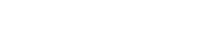2. Find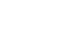3. Let us take the function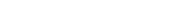When x = 4, is the function increasing or decreasing? Is the function concave up, or concave down?

4. A ball is thrown. The height of the ball in meters at any given time can be found by the function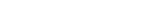where t is in seconds.

a) At what moment has the ball reached its highest point?
b) What is the acceleration of the ball at this point?

5. A particle moves in a straight line with the velocity given by the equation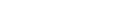What is the total displacement between 0 seconds and 4 seconds?

6.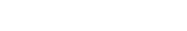Find r.

7. Find the area enclosed by the graphs of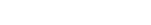Solutions:

1.2. If we plug in x=0 into the equation, we get a 0 in the denominator. If the expression had evaluated to a real number, the problem would be done. This is not the case here.
Our best approach would be to use L’Hopital’s Rule. Take the derivative of the top and bottom, and then evaluate the integral.3. To find where a function increases or decreases, we need to take the derivativeAt x = 4, this evaluates to 26, so this function is increasing at that point.

To determine concavity, let’s take the second derivativeTherefore, the function is concave up. Since we could look at the initial equation and see that it is an upward facing parabola, we could answer this part without actually taking the derivative but rather by knowing that a parabola’s concavity is always either upward and downward (depending on which way the parabola faces.)

4. To find the moment the ball reaches it highest point, we are looking for the moment the velocity is zero. To find this, take the derivative at set it to 0.The ball reaches its highest point at t = 4/10 seconds.

The acceleration of the ball at this point is the double derivative of this equation.The acceleration is a constant -10 m/s.

5. The displacement of a particle can be found by evaluating the integral between the two times given.This evaluates to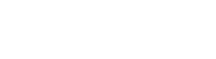6.

We need to evaluate the integralTherefore, if we solve this, we find that r = 1.

7.

Anytime we are looking for the area under a curve, we are looking for an indefinite integral. However, in this case, we also need to know the end points. This is where the two graphs cross.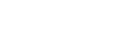we find that x = 0 or x = 1, our endpoints.Improve your SAT or ACT score, guaranteed. Start your 1 Week Free Trial of Magoosh SAT Prep or your 1 Week Free Trial of Magoosh ACT Prep today!Author

•Zachary is a former mechanical engineer and current high school physics, math, and computer science teacher. He graduated from McGill University in 2011 and spent time in the automotive industry in Detroit before moving into education. He has been teaching and tutoring for the past five years, but you can also find him adventuring, reading, rock climbing, and traveling whenever the opportunity arises.